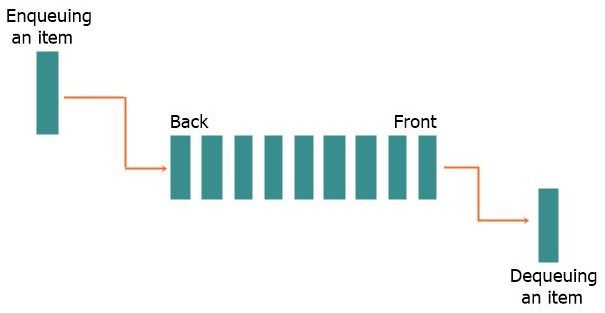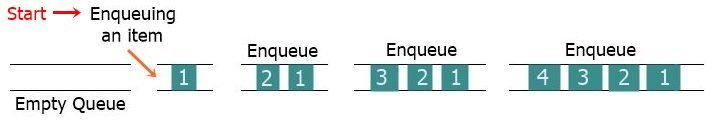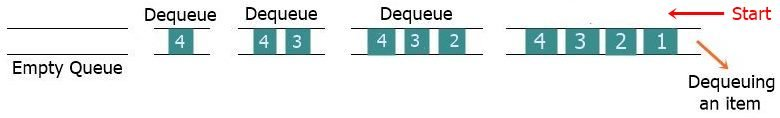# Queue in Data Structure

One of the most well-known, speedy, and adaptable data structures is the "queue," which will be discussed in this article. Let's get right down to the introductory section then.

## Introduction to Queue Data Structure

To put it simply, a queue is a linear data structure because it stores information in a sequential order. The following image illustrates the concept of "queue" by demonstrating how information is structured within it.Each rectangular line in the above pictorial representation of a queue represents one item of the queue, with the first item from the front being the item that was inserted into the queue first and will come out first, and the last item from the back being the item that will come out last.

The term "back" can also be replaced with "rear," because some programmers believe that the back of a "queue" data structure falls into the category of "rear" items.

## The queue follows the FIFO principle

The queue operates on the first-in, first-out (FIFO) principle. The "queue" data structure follows the First-In-First-Out (FIFO) principle, which states that the queue elements inserted first are the first to come out. Consider the crowds gathered to board a bus, train, or other mode of transportation. The person who started the line will be the first to get the ticket and exit the line, and the person who joins the line at the very end will be the last to get the ticket and exit the line.

When the last person leaves the queue, it will be empty.

In the programming world, because queue follows the first-in, first-out principle, it will be useful where processes must be performed in an ordered manner. Consider the following scenarios:

• Typically, queues are used as waiting lists for a single shared resource, such as a printer, disc, or central processing unit (CPU).
• Pipes, file I/O, and sockets are all examples of asynchronous data transfer, in which data is not passed back and forth between processes at the same rate, therefore "queue" is used there.
• Media players use queues to keep track of their play lists, allowing users to add and remove songs as needed.
• Operating systems use queues for interrupt management.
• and so on.

## Queue Operations

Similar to the "stack,"  the queue data structure also performs the two operations, which are as follows:

• "Enqueue": the insertion of an item into the queue.
• "Dequeue": the removal or deletion of an item from the queue.

In other words, adding a new item to the queue is referred to as "enqueue," whereas removing an item from the queue is referred to as "dequeue." The "enqueue" operation is performed at the end (rear) of the queue, whereas the "dequeue" operation is performed at the beginning (front) of the queue.

Let me now draw a visual representation of how items in a queue are enqueued. Now, I've created a graphical representation of items being enqueued into a queue, which is shown below:That is, I started with an empty queue, then enqueued (inserted) an item into it, then inserted another item into it, and so on. Now, if you want to dequeue an item from the queue, the item will be dequeued from the front; thus, based on the above queue representation, the "1" will be the first item to be removed if we dequeue an item from the queue.

To help you understand the concept, let me draw another graphical representation of how the dequeue operation occurs in the queue data structure.That is, I inserted four items into the queue using the same queue that was created after four enqueue operations. To demonstrate dequeue, I dequeue four times in the above figure to empty the queue. When I dequeue, the first item to be removed is "1," because it is at the top of the queue; then "2," and so on. Queue data structure works in this manner.

Data Structure Quiz

« Previous Tutorial CodesCracker »